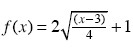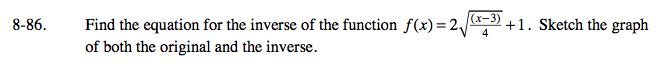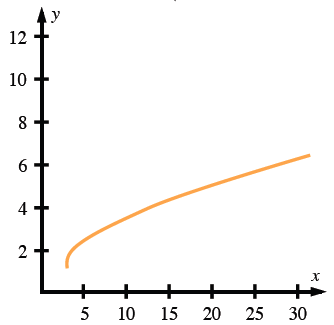Home > A2C > Chapter 8 > Lesson 8.1.5 > Problem8-86

8-86.

Find the equation for the inverse of the functionSketch the graph of both the original and the inverse. Homework Help ✎To find the inverse, change every x to f(x) and every f(x) to x. Solve for f(x).

If you found a few points for the graph of f(x), how can that help you graph the inverse equation?